The Spheres ClipArt gallery includes 74 images of spheres, including parts and zones of spheres and hemispheres. A sphere is a 3-dimensional figure in which all points are equidistant from a center point.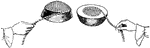### Area of Sphere

"It is found that the cord covering the curved surface is twice as long as the one covering the flat…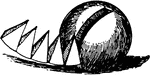### Area of Sphere

"The bases of the pyramids are considered as forming the surface of the sphere, while the altitude of…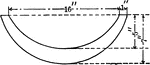### Vertical Cross Section of Spherical Zones of a Casting

An illustration of a vertical cross section of the spherical zones of a casting with a diameter of 16…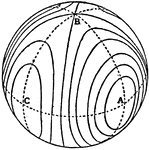### Invariable Cone

Possible forms of the invariable cone by means of the intersections with a concentric spherical surface.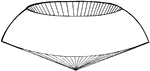### Spherical Cone

"If a sector revolves about its bounding radius as an axis, it generates what may be called a spherical…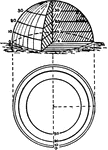### Contours of a half sphere

"The contours of a half sphere are a series of circles, far apart near the center (top) and near together…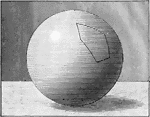### Spherical Polygon

Illustration of a spherical polygon.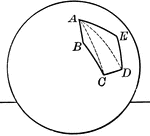### Spherical Polygon

Illustration of a spherical polygon.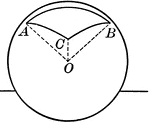### Spherical Pyramid

Illustration of a spherical pyramid.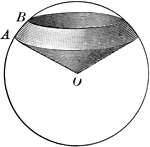### Spherical Sector

Illustration of a spherical sector.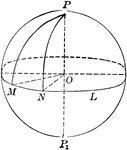### Sphere With Sectors and Arcs

Diagram of a sphere with sectors and arcs drawn.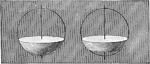### Spherical Segments

Illustration of a spherical segments.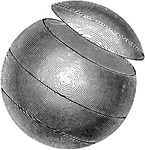### Small Circle

A small circle is one formed by a plane which does not cut the earth into two equal parts. The small…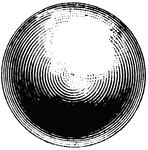### Sphere

A sphere is a solid bounded by a uniformly curved surface, every point of which is equally distant from…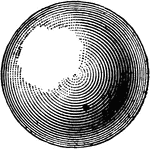### Sphere

"A sphere is a solid bounded by a uniformly curved surface, every point of which is equally distant…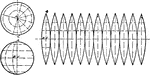### Development of Sphere Gore Method

An illustration in flattening the sphere using Gore method by creating cylinder sections with equal…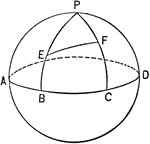### Sphere With Sectors and Arcs

Illustration of a sphere with sectors and arcs.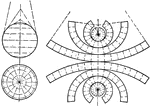### Development of Sphere Zone Method

An illustration of a development of sphere using Zone method by creating sections of rolled out cones.### Sphere With 8-inch Diameter Cut by Planes

An 8-inch sphere cut by parallel planes, one 2 inches from center and the other 6 inches from center.…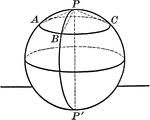### Sphere With Arcs and Poles

A sphere with arcs and poles. "The distance of all points in the circumference of a circle of a sphere…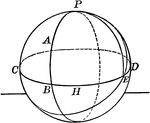### Arcs Drawn on Sphere

Illustration of a sphere with arcs drawn.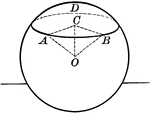### Circle Made by Plane Passing Through a Sphere

"Every section of a sphere made by a plane is a circle."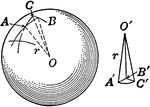### Sphere Cut Into Pyramids.

An illustration of a sphere cut into polygons as bases with their vertices at the center of sphere.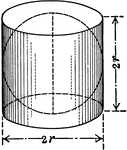### Sphere Inside of Cylinder.

An illustration of a sphere inside of a cylinder with radius/diameter labeled. Illustration for showing…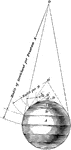### Development Of Sphere

Illustration showing the development of a sphere by the perspective of its resolved cones.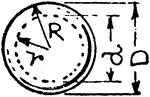### Hollow Sphere

An illustration of a hollow sphere.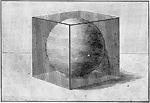### Sphere Inscribed in a Cube

Illustration of a sphere inscribed in a cube.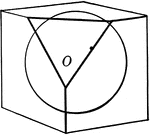### Sphere Inscribed in a Cube

Illustration of a sphere inscribed in a cube.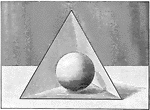### Sphere Inscribed in Tetrahedron

Illustration of a sphere inscribed in a tetrahedron.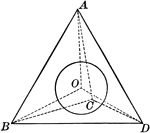### Sphere Inscribed in Tetrahedron

Illustration of a sphere inscribed in a tetrahedron.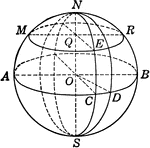### Sphere with arcs and circles labeled.

An illustration of a sphere showing diameter, arcs, and circles. A sphere is a solid bounded by a curved…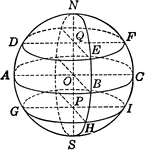### Sphere With Arcs, Circles, and Zones Labeled.

An illustration of a sphere showing diameter, arcs, and circles. A sphere is a solid bounded by a curved…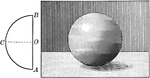### Sphere Made by Revolution of Semicircle

Illustration of a sphere generated by the revolution of a semicircle ACB about its diameter AB as an…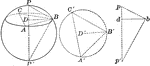### Material Sphere

Illustration of a material sphere that can be used to find its diameter.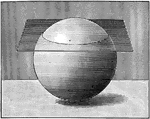### Plane Passing Through a Sphere

Illustration of a plane passing through a sphere.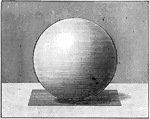### Sphere With Tangent Plane

Illustration of a sphere with a tangent plane. "A plane perpendicular to a radius at its extremity is…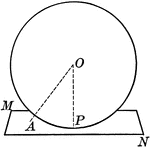### Sphere With Tangent Plane

Illustration of a sphere with a tangent plane. "A plane perpendicular to a radius at its extremity is…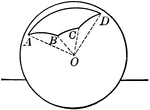### Polyhedral Angle in a Sphere

Illustration of a sphere with a polyhedral angle whose vertex is at the center of the sphere and whose…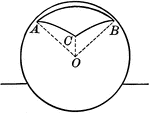### Polyhedral Angle in a Sphere

Illustration of a sphere with a polyhedral angle.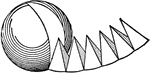### Sphere Formed By Pyramids

Illustration showing that "the solids formed by the dissected part of the sphere are pyramids."### Sphere With Quadrants and Poles

A sphere with quadrants and poles. "A point on the surface of a sphere, which is at the distance of…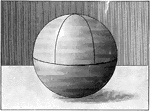A sphere with quadrants drawn on it.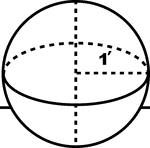### Sphere With a Radius of 1 foot

An illustration of a sphere showing a radius of 1 foot. Illustration could be used for calculating volume.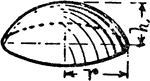### Segment Of Sphere

An illustration of a segment of a sphere.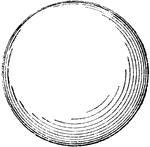### Wooden Sphere

A wooden hollow sphere created by using a lathe and wooden templet at a desired diameter.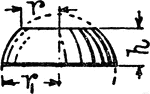### Zone Of Sphere

An illustration of a zone of a sphere.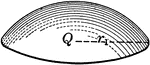### Zones or Segments of Spheres

An illustration of a zone of a sphere. A zone occurs when a sphere is cut by parallel planes that are…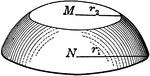### Zones or Segments of Spheres

An illustration of a zone of a sphere. A zone occurs when a sphere is cut by parallel planes that are…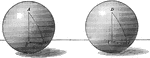### Equal Spheres With Triangles

Illustration of equal spheres with equal triangles.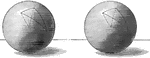### Equal Spheres With Triangles

Illustration of equal spheres with equilateral and equiangular triangles.### Intersecting Spheres

Illustration of two intersecting spheres. "The intersection of two spherical surfaces is the circumference…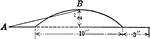### Flanged Spherical Segment

An illustration of a flanged spherical segment with a diameter of 10 inches. Illustration could be used…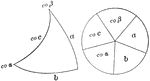### Napier's Right Spherical Triangle

Illustration of a right spherical triangle and the five circular parts placed in the sectors of a circle…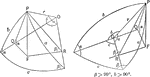### Relationships In A Spherical Triangle

Illustration used, with the law of sines, to find the relation between two sides of a spherical triangle…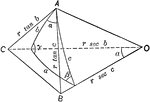### Relationships In A Spherical Triangle

Illustration used, with the law of cosines, to find the relation between the three sides and an angle…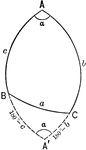### Relationships In A Spherical Triangle

Illustration used to extend the law of cosines when finding the relation between the three sides and…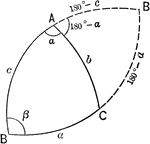### Relationships In A Spherical Triangle

Illustration used to extend the law of cosines when finding the relation between the three sides and…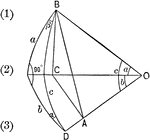### Right Spherical Triangle

Illustration of a right spherical triangle with a and b the sides, and α and β the angles…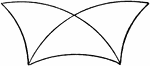### Superimposed Symmetrical Spherical Triangles

Diagram showing 2 superimposed symmetrical spherical triangles.